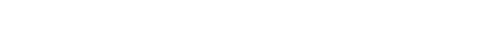glOrtho.3glOpenGL man pages

```

```

## Name

```  glOrtho - multiply the current matrix	by an orthographic matrix

```

## C Specification

```  void glOrtho(	GLdouble left,
GLdouble right,
GLdouble bottom,
GLdouble top,
GLdouble near,
GLdouble far )

```

## Parameters

```
left,	right Specify the coordinates for the left and right vertical
clipping planes.

bottom, top Specify the coordinates for the bottom and top horizontal
clipping planes.

near,	far   Specify the distances to the nearer and farther depth clipping
planes.  These distances are negative if the plane is to be
behind the viewer.

```

## Description

```  glOrtho describes a perspective matrix that produces a parallel projection.
(left, bottom,  -near) and (right, top,  -near) specify the points on	the
near clipping	plane that are mapped to the lower left	and upper right
corners of the window, respectively, assuming	that the eye is	located	at
(0, 0, 0).  -far specifies the location of the far clipping plane.  Both
near and far can be either positive or negative.  The	corresponding matrix
is

|     2	     		          |
|----------      0          0      t  |
|right-left				x |
|					  |
|		      2    		  |
|    0       ----------	0      t  |
|	  	 top-bottom	        y |
|                                     |
|					  |
|	 0	     0	        -2        |
|                        --------  t  |
|			     far-near	z |
|                                     |
|	 0	     0		0      1  |

where
right+left
t  = - ----------
x     right-left

top+bottom
t  = - ----------
y     top-bottom

far+near
t  = - --------
z     far-near

The current matrix is	multiplied by this matrix with the result replacing
the current matrix.  That is,	if M is	the current matrix and O is the	ortho
matrix, then M is replaced with M · O.

Use glPushMatrix and glPopMatrix to save and restore the current matrix
stack.

```

## Errors

```  GL_INVALID_OPERATION is generated if glOrtho is executed between the
execution of	glBegin	and the	corresponding execution	of glEnd.

```

## Associated Gets

```  glGet	with argument GL_MATRIX_MODE
glGet	with argument GL_MODELVIEW_MATRIX
glGet	with argument GL_PROJECTION_MATRIX
glGet	with argument GL_TEXTURE_MATRIX

```

```  glFrustum, glMatrixMode, glMultMatrix, glPushMatrix, glViewport